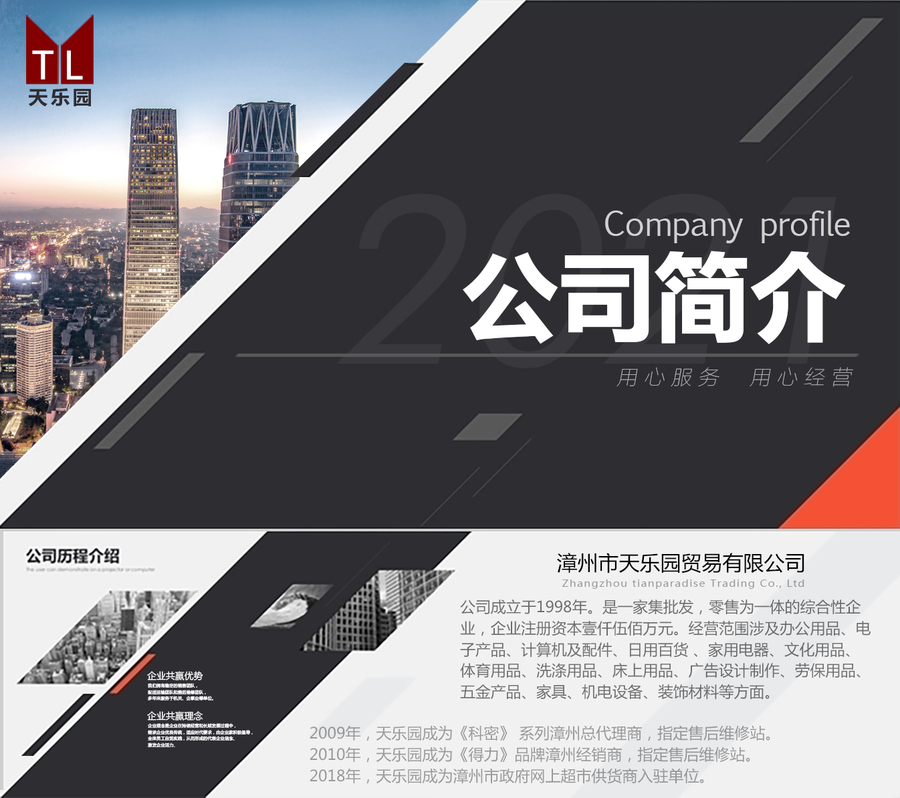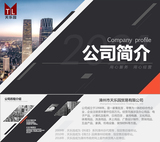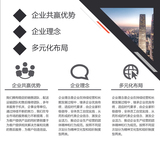• 品牌
得力
科密
天章
戴尔
联想
华硕
宏基
清华同方
惠普
易昌
海尔
乐事
微软
华为
海尔
惠普
佳能
飞利浦
松下
尼康
• 计算机设备及软件
计算机设备
台式计算机
便携式计算机
服务器
平板式微型计算机
计算机网络设备
路由器
以太网交换机
信息安全设备
防火墙
入侵检测设备
入侵防御设备
漏洞扫描设备
容灾备份设备
网络隔离设备
安全审计设备
网闸
网上行为管理设备
终端设备
触摸式终端设备
终端机
存储设备
磁盘阵列
存储用光纤交换机
光盘库
输入输出设备
打印设备
喷墨打印机
激光打印机
针式打印机
热式打印机
显示设备
液晶显示器
刷卡机
触摸屏
图形图像输入设备
扫描仪
识别输入设备
机房辅助设备
机柜
计算机软件
操作系统
办公套件
• 办公数码设备
办公设备
投影仪
投影幕
多功能一体机
照相机及器材
数字照相机
镜头及器材
电子白板
LED显示屏
触控一体机
刻录机
打印机
文印设备
装订机
速印机
销毁设备
碎纸机
光盘粉碎机
• 条码打印机
• 条码扫描器
• 生活用电器
制冷电器
电冰箱
冷藏柜
空气调节电器
风扇
通风机
空调机
• 通信设备
电话通信设备
普通电话机
特种电话机
移动电话
视频会议系统设备
视频会议多点控制器（MCU）
视频会议会议室终端
视频会议控制台
音视频矩阵
• 广播电视电影设备
电视设备
普通电视设备（电视机）
视频设备
通用摄像机
• 办公消耗用品
复印纸/打印纸
• 办公设备
点钞机
考勤机
真空包装机
塑封机
切纸机
监控摄像头
指纹锁
包装机耗材
喷码机
• 计算器
语音型计算器
太阳能双电源计算器
科学函数型计算器
桌上型计算器
• 本册纸品
打印/复印纸
软面抄系列
皮面抄系列
螺旋本
作文本/英语本/纠错本
文艺小清新本册
手账本
乐素系列本册
皮卡丘系列本册
美术本册
单据/账本账册
百事贴/N次贴
自粘性标贴
便签本/草稿纸
信封/信纸
活页替芯
荣誉证书
硬抄本
• 保险箱/柜
小型保险箱
中型保险箱
3C保险箱
• 会议用品
白板及配件
翻页笔
投影幕布
液晶电子手写板
国旗
荣誉证书
桌牌/磁性标贴
转换器
• 桌面文具
美工刀
票夹
笔筒
三针一钉
仪尺
文件座/文件框
文件柜
印台/油印
固体胶/液体胶
打孔机
书立
剪刀
订书机
橡胶圈
点点胶
切割垫板
• 收纳整理
牛皮纸收纳盒
桌面收纳
• 文件管理
装订夹条
文件夹
资料册
快劳夹
拉链袋
票据包/夹
风琴包/事物包
文件套/文件袋
档案盒/袋
名片册/名片座 /名片包
板夹/复写板
证件套
抽杆文件夹
收纳袋/公文包
• 书写系列
中性笔
圆珠笔
荧光笔
记号笔
白板笔
铅笔
笔替芯
钢笔
台笔
活动铅笔
• 办公打印
热敏打印纸
传真纸系列
硒鼓
收银纸
复写纸
碳带
• 白板封装
白板
白板配件
胶带
封箱器
胶带座
• 学生文具
学生书写
卷笔刀/削笔机
修正带/橡皮擦
文具盒/笔袋
文具礼盒
学习工具
绘画用品
益智系类
书皮书套
学生手工
书包
• 颐和园系列
幻彩霓裳
清风拂月
楼阁书香
• 办公电子
计算器
激光笔
电话机
电源插座
鼠标键盘
存储/充电宝
电池
• 办公生活用品
报刊架/杂志架
地球仪
小风扇
号码机
标价机
挂钟/闹钟
台灯
温湿度计
清洁用品
工具
休闲娱乐
自封塑料袋/封口袋
钥匙管理箱
发热桌垫/鼠标垫
保温杯/壶
水杯/纸杯
体重称/健康称
电热水壶
办公椅
• 胶黏用品
胶带
绵纸胶带
和纸胶带
胶水
胶棒
强力胶
• 热敏
• 装订
• 高拍仪
• 步步高 W202 子母电话机
• 科密碎纸机
• 海尔空调机
• 震旦一体机
• 震旦激光打印机
• 震旦
• 惠普打印机
• 震旦复印机
• 爱普生投影仪
• 柯尼卡美能达激光打印机
• 兄弟激光打印机
• 索尼摄像机
• 联想笔记本
• 联想打印机
• 得实针式打印机
• 得实标签打印机
• 索尼投影仪
• 奔图激光打印机
• 奔图多功能一体机
• 得力条码打印机
• 爱普生喷墨打印机
• 柯尼卡美能达多功能一体机
• 科密装订机
• 京瓷多功能一体机
• 长虹电视机
• 创维电视机
• 格力空调机
• 爱普生针式打印机
• 康佳电视机
• 兄弟多功能一体机
• 艾美特电风扇
• 百事达复印纸
• 尖兵复印纸
• 清风复印纸
• 晶纯复印纸
• 天章复印纸
• 得力装订机
• 联想台式电脑
• 理想速运机
• 理想速印机
• 科密条码扫描器
• 海尔电冰箱
• 美的电风扇
• 晨光碎纸机
• 金典碎纸机
• 金典装订机
• 汉王扫描仪
• 爱普生扫描仪
• 紫光扫描仪
• 联想服务器
• 惠普激光打印机
• 松下摄像机
• 得实热式打印机
• 飞利浦电视机
• 得力A3复印纸
• 得力复印纸
• 华硕台式计算机
• 华硕笔记本
• 天章韵复印纸
• 美的冰柜
• 华为平板式笔记本
• 惠普台式电脑
• 步步高电话机
• 得力电话机
• 华为移动电话
• 戴尔液晶显示器
• 齐心复印纸
• 海尔冷藏柜
• 佳能照相机
• 索尼照相机
• 尼康照相机
• 得力扫描枪
• 数码照相机
• 得力碎纸机
• 惠普液晶显示器
• 起航
• 容声
• 美的
• 三木
• 盆景
• 三菱重工
• 美的空调机
• 京瓷
• 美的电冰箱
• 戴尔服务器
• 创维触控一体机
• 松下投影仪
• 起航学生考卷专用纸
• 美的中央空调机
• 海尔电视机
• 理光
• 实达
• 爱普生
• 奔图
• 映美
• 容声冷藏柜
• 容声电冰箱
• 汉王
• 良田
• 齐心
• 歌派
• 富士施乐
• 柯尼卡美能达一体机
• 夏普
• 乐活天章复印纸
• 华为路由器
• 锐捷
• 华三
• 普联
• 海康威视
• 中控智慧
• 索尼
• 高品乐
• 晨光
• 百旺
• 思路
• 世纪天章
• 乐活天章
• 艾美特
• 晨鸣
• 柯尼卡美能达
• 雅欣
• 步步高
• 格力
• 得实
• 理想之星
• 创维
• 百事达
• 兄弟
• 志高
• 金典
• 康佳
• 斑马
• 奥克斯
• 东方中原
• 海信
• 宝泰尔
• 中诺
• 未来世界
• 荣大
• 派克斯
• 紫光
• 雅文
• 希沃
• 美宝视
• 明基
• 鸿合
• 艾博德
• 富士通
• 麦克赛尔
• 长虹
• 标拓
• 基士得耶
• 艾泰
• 每思会
• 柯达
• 中航信息
• 冠捷
• 爱胜品
• 东芝
• 佳能通用摄像机
• 东方中原触摸一体机
• 海信电视机
• 投影布
• 复印纸
• 冲电气
• 方正
• 极米
• 汇金
• 电视机
• 华为手机
• 王牌电视机
• 笔记本电脑
• 坚果
• 霍尼韦尔
• 紫图
• 利盟
• 富士
• 智本
• 碎乐
• 理想
• 银佳
• 友讯
• 和冠
• 光电通
• 利亚德
• 索诺克
• 宏碁
• 恩益禧
• 恩益禧/NEC NP-CR3126 投影仪
• 优派
• 光峰
• 伟文
• 四通
• 互视达
• 新浅
• 皓丽
• 汉光
• 新线
• 三星
• 中晶
• 点易拍
• 影源
• 精益
• 图腾
• 科大讯飞
• 杰必喜
• 雷盛
• 浩顺
• 讯宝
• 红叶
• 天雄巨彩
• 理光复印机
• 夏普复印机
• 柯尼美能达
• 浪潮
• 图腾·
• 他抬头
• 科视
• 海佳彩亮
• 新都
• 深信服
• 讯光
• 成者
• 荣耀
• 三叶
• 强力巨彩
• 裕佳
• 杰美特
• 麦克
• 安恒
• 京1瓷
• 惠普电脑
• 金翔
• 蓝普LED屏
• 沧田
• 仙视
• 得力绿柏复印纸
• 步卡止复印纸
• 得力打印机
• 惠普扫描仪
• 得力多功能一体机
• 创显
• 升腾
• 帝道
• 清华
• 沃开
• 科创
• 和谐
• 王牌
• 捷宇
• 启沃
• 莱特斯
• 中兴
• 长城
• 海峡彩亮
• 筑影
• 大华
• 奥图码
• 爱普伦
• 欧帝
• 佳文
• 华美
• 道顿
• 联想彩色打印机
• 王牌冰箱
• 汉光多功能一体机
• 鑫耀
• 新视觉
• 晶彩
• 人脸考勤机
• 惠普台式计算机
• 联想·
• Alzhihan
• 立象
• 新的
• 方正国际
• 蓝普
• 先尚
• 汉光创联
• 迪普乐
• 京东方
• 德凡
• 立思辰
• 中银
• 联系
• 赫斯密
• 奥士达
• AGM
• 山特
• 惠朗
• 欧市利
• 卓越佳印
• 金铭洋
• 安普印
• 博思得
• 中矗
• 宜联
• 下朴素
• 德玛仕
• 不间断电源
• 山克
• 麦克塞尔
• 阿芙罗
• 多利
• 雷鸟
• 爱国者
• 联建光电
• 富士胶片
• 美的风扇
• 兄弟条码打印机
• 夏普多功能一体机
• 华为便携式计算机
• 碎乐碎纸机
• 戴尔台式计算机
• 联想便携式计算机
• 山特不间断电源
• 海康威视台式计算机
• 恩益禧投影仪
• 明基投影仪
• 光电通激光打印机
• 紫光台式计算机
• 晨光刷卡机
• 得力激光打印机
• 联想台式计算机
• 惠普便携式计算机
• 理光多功能一体机
• 海康威视显示屏
• 海康威视液晶显示屏
• 海康威视液晶显示器
• 海康威视服务器
• 海康威视磁盘阵列
• 海康威视触控一体机
• 海康威视音视频矩阵
• 海康威视刷卡机
• 华为平板式微型计算机
• 华为台式计算机
• 华为电视机
• 得实条码打印机
• 鸿合触控一体机
• 联想激光打印机
• 海信电冰箱
• 海信空调机
• 海信冷藏柜
• 海信投影仪
• 海信触控一体机
• 蓝普LED显示屏
• 创维普通电视设备（电视机）
• 创维液晶显示器
• 世纪天章复印纸
• 经典佳印复印纸
• 世纪佳印
• 晨鸣丽雅复印纸
• 荣耀移动电话
• 方正多功能一体机
• 艾美特风扇
• 鑫永佳复印纸
• 汉王刷卡机
• 北信源信息安全软件
• 阿芙罗复印纸
• 联想平板式微型计算机
• 联想触控一体机
• 联想多功能一体机
• 强力巨彩LED显示屏
• 海信LED显示屏
• 海信液晶显示器
• 海康威视视频会议会议室终端
• 海康威视LED显示屏
• 小钢炮
• 金旗舰复印纸
• 未来世界复印纸
• 小钢炮复印纸
• 金丝雀复印纸
• 酷酷虫复印纸
• 益思复印纸
• 优仕复印纸
• 冲击波复印纸
• 如佳复印纸
• 多利复印纸
• 文印复印纸
• 希沃触控一体机
• 震旦多功能一体机
• 天章龙复印纸
• 美菱电冰箱
• 朗印复印纸

• 品牌
得力
科密
天章
戴尔
联想
华硕
宏基
清华同方
惠普
易昌
海尔
乐事
微软
华为
海尔
惠普
佳能
飞利浦
松下
尼康
• 计算机设备及软件
计算机设备
台式计算机
便携式计算机
服务器
平板式微型计算机
计算机网络设备
路由器
以太网交换机
信息安全设备
防火墙
入侵检测设备
入侵防御设备
漏洞扫描设备
容灾备份设备
网络隔离设备
安全审计设备
网闸
网上行为管理设备
终端设备
触摸式终端设备
终端机
存储设备
磁盘阵列
存储用光纤交换机
光盘库
输入输出设备
打印设备
喷墨打印机
激光打印机
针式打印机
热式打印机
显示设备
液晶显示器
刷卡机
触摸屏
图形图像输入设备
扫描仪
识别输入设备
机房辅助设备
机柜
计算机软件
操作系统
办公套件
• 办公数码设备
办公设备
投影仪
投影幕
多功能一体机
照相机及器材
数字照相机
镜头及器材
电子白板
LED显示屏
触控一体机
刻录机
打印机
文印设备
装订机
速印机
销毁设备
碎纸机
光盘粉碎机
• 条码打印机
• 条码扫描器
• 生活用电器
制冷电器
电冰箱
冷藏柜
空气调节电器
风扇
通风机
空调机
• 通信设备
电话通信设备
普通电话机
特种电话机
移动电话
视频会议系统设备
视频会议多点控制器（MCU）
视频会议会议室终端
视频会议控制台
音视频矩阵
• 广播电视电影设备
电视设备
普通电视设备（电视机）
视频设备
通用摄像机
• 办公消耗用品
复印纸/打印纸
• 办公设备
点钞机
考勤机
真空包装机
塑封机
切纸机
监控摄像头
指纹锁
包装机耗材
喷码机
• 计算器
语音型计算器
太阳能双电源计算器
科学函数型计算器
桌上型计算器
• 本册纸品
打印/复印纸
软面抄系列
皮面抄系列
螺旋本
作文本/英语本/纠错本
文艺小清新本册
手账本
乐素系列本册
皮卡丘系列本册
美术本册
单据/账本账册
百事贴/N次贴
自粘性标贴
便签本/草稿纸
信封/信纸
活页替芯
荣誉证书
硬抄本
• 保险箱/柜
小型保险箱
中型保险箱
3C保险箱
• 会议用品
白板及配件
翻页笔
投影幕布
液晶电子手写板
国旗
荣誉证书
桌牌/磁性标贴
转换器
• 桌面文具
美工刀
票夹
笔筒
三针一钉
仪尺
文件座/文件框
文件柜
印台/油印
固体胶/液体胶
打孔机
书立
剪刀
订书机
橡胶圈
点点胶
切割垫板
• 收纳整理
牛皮纸收纳盒
桌面收纳
• 文件管理
装订夹条
文件夹
资料册
快劳夹
拉链袋
票据包/夹
风琴包/事物包
文件套/文件袋
档案盒/袋
名片册/名片座 /名片包
板夹/复写板
证件套
抽杆文件夹
收纳袋/公文包
• 书写系列
中性笔
圆珠笔
荧光笔
记号笔
白板笔
铅笔
笔替芯
钢笔
台笔
活动铅笔
• 办公打印
热敏打印纸
传真纸系列
硒鼓
收银纸
复写纸
碳带
• 白板封装
白板
白板配件
胶带
封箱器
胶带座
• 学生文具
学生书写
卷笔刀/削笔机
修正带/橡皮擦
文具盒/笔袋
文具礼盒
学习工具
绘画用品
益智系类
书皮书套
学生手工
书包
• 颐和园系列
幻彩霓裳
清风拂月
楼阁书香
• 办公电子
计算器
激光笔
电话机
电源插座
鼠标键盘
存储/充电宝
电池
• 办公生活用品
报刊架/杂志架
地球仪
小风扇
号码机
标价机
挂钟/闹钟
台灯
温湿度计
清洁用品
工具
休闲娱乐
自封塑料袋/封口袋
钥匙管理箱
发热桌垫/鼠标垫
保温杯/壶
水杯/纸杯
体重称/健康称
电热水壶
办公椅
• 胶黏用品
胶带
绵纸胶带
和纸胶带
胶水
胶棒
强力胶
• 热敏
• 装订
• 高拍仪
• 步步高 W202 子母电话机
• 科密碎纸机
• 海尔空调机
• 震旦一体机
• 震旦激光打印机
• 震旦
• 惠普打印机
• 震旦复印机
• 爱普生投影仪
• 柯尼卡美能达激光打印机
• 兄弟激光打印机
• 索尼摄像机
• 联想笔记本
• 联想打印机
• 得实针式打印机
• 得实标签打印机
• 索尼投影仪
• 奔图激光打印机
• 奔图多功能一体机
• 得力条码打印机
• 爱普生喷墨打印机
• 柯尼卡美能达多功能一体机
• 科密装订机
• 京瓷多功能一体机
• 长虹电视机
• 创维电视机
• 格力空调机
• 爱普生针式打印机
• 康佳电视机
• 兄弟多功能一体机
• 艾美特电风扇
• 百事达复印纸
• 尖兵复印纸
• 清风复印纸
• 晶纯复印纸
• 天章复印纸
• 得力装订机
• 联想台式电脑
• 理想速运机
• 理想速印机
• 科密条码扫描器
• 海尔电冰箱
• 美的电风扇
• 晨光碎纸机
• 金典碎纸机
• 金典装订机
• 汉王扫描仪
• 爱普生扫描仪
• 紫光扫描仪
• 联想服务器
• 惠普激光打印机
• 松下摄像机
• 得实热式打印机
• 飞利浦电视机
• 得力A3复印纸
• 得力复印纸
• 华硕台式计算机
• 华硕笔记本
• 天章韵复印纸
• 美的冰柜
• 华为平板式笔记本
• 惠普台式电脑
• 步步高电话机
• 得力电话机
• 华为移动电话
• 戴尔液晶显示器
• 齐心复印纸
• 海尔冷藏柜
• 佳能照相机
• 索尼照相机
• 尼康照相机
• 得力扫描枪
• 数码照相机
• 得力碎纸机
• 惠普液晶显示器
• 起航
• 容声
• 美的
• 三木
• 盆景
• 三菱重工
• 美的空调机
• 京瓷
• 美的电冰箱
• 戴尔服务器
• 创维触控一体机
• 松下投影仪
• 起航学生考卷专用纸
• 美的中央空调机
• 海尔电视机
• 理光
• 实达
• 爱普生
• 奔图
• 映美
• 容声冷藏柜
• 容声电冰箱
• 汉王
• 良田
• 齐心
• 歌派
• 富士施乐
• 柯尼卡美能达一体机
• 夏普
• 乐活天章复印纸
• 华为路由器
• 锐捷
• 华三
• 普联
• 海康威视
• 中控智慧
• 索尼
• 高品乐
• 晨光
• 百旺
• 思路
• 世纪天章
• 乐活天章
• 艾美特
• 晨鸣
• 柯尼卡美能达
• 雅欣
• 步步高
• 格力
• 得实
• 理想之星
• 创维
• 百事达
• 兄弟
• 志高
• 金典
• 康佳
• 斑马
• 奥克斯
• 东方中原
• 海信
• 宝泰尔
• 中诺
• 未来世界
• 荣大
• 派克斯
• 紫光
• 雅文
• 希沃
• 美宝视
• 明基
• 鸿合
• 艾博德
• 富士通
• 麦克赛尔
• 长虹
• 标拓
• 基士得耶
• 艾泰
• 每思会
• 柯达
• 中航信息
• 冠捷
• 爱胜品
• 东芝
• 佳能通用摄像机
• 东方中原触摸一体机
• 海信电视机
• 投影布
• 复印纸
• 冲电气
• 方正
• 极米
• 汇金
• 电视机
• 华为手机
• 王牌电视机
• 笔记本电脑
• 坚果
• 霍尼韦尔
• 紫图
• 利盟
• 富士
• 智本
• 碎乐
• 理想
• 银佳
• 友讯
• 和冠
• 光电通
• 利亚德
• 索诺克
• 宏碁
• 恩益禧
• 恩益禧/NEC NP-CR3126 投影仪
• 优派
• 光峰
• 伟文
• 四通
• 互视达
• 新浅
• 皓丽
• 汉光
• 新线
• 三星
• 中晶
• 点易拍
• 影源
• 精益
• 图腾
• 科大讯飞
• 杰必喜
• 雷盛
• 浩顺
• 讯宝
• 红叶
• 天雄巨彩
• 理光复印机
• 夏普复印机
• 柯尼美能达
• 浪潮
• 图腾·
• 他抬头
• 科视
• 海佳彩亮
• 新都
• 深信服
• 讯光
• 成者
• 荣耀
• 三叶
• 强力巨彩
• 裕佳
• 杰美特
• 麦克
• 安恒
• 京1瓷
• 惠普电脑
• 金翔
• 蓝普LED屏
• 沧田
• 仙视
• 得力绿柏复印纸
• 步卡止复印纸
• 得力打印机
• 惠普扫描仪
• 得力多功能一体机
• 创显
• 升腾
• 帝道
• 清华
• 沃开
• 科创
• 和谐
• 王牌
• 捷宇
• 启沃
• 莱特斯
• 中兴
• 长城
• 海峡彩亮
• 筑影
• 大华
• 奥图码
• 爱普伦
• 欧帝
• 佳文
• 华美
• 道顿
• 联想彩色打印机
• 王牌冰箱
• 汉光多功能一体机
• 鑫耀
• 新视觉
• 晶彩
• 人脸考勤机
• 惠普台式计算机
• 联想·
• Alzhihan
• 立象
• 新的
• 方正国际
• 蓝普
• 先尚
• 汉光创联
• 迪普乐
• 京东方
• 德凡
• 立思辰
• 中银
• 联系
• 赫斯密
• 奥士达
• AGM
• 山特
• 惠朗
• 欧市利
• 卓越佳印
• 金铭洋
• 安普印
• 博思得
• 中矗
• 宜联
• 下朴素
• 德玛仕
• 不间断电源
• 山克
• 麦克塞尔
• 阿芙罗
• 多利
• 雷鸟
• 爱国者
• 联建光电
• 富士胶片
• 美的风扇
• 兄弟条码打印机
• 夏普多功能一体机
• 华为便携式计算机
• 碎乐碎纸机
• 戴尔台式计算机
• 联想便携式计算机
• 山特不间断电源
• 海康威视台式计算机
• 恩益禧投影仪
• 明基投影仪
• 光电通激光打印机
• 紫光台式计算机
• 晨光刷卡机
• 得力激光打印机
• 联想台式计算机
• 惠普便携式计算机
• 理光多功能一体机
• 海康威视显示屏
• 海康威视液晶显示屏
• 海康威视液晶显示器
• 海康威视服务器
• 海康威视磁盘阵列
• 海康威视触控一体机
• 海康威视音视频矩阵
• 海康威视刷卡机
• 华为平板式微型计算机
• 华为台式计算机
• 华为电视机
• 得实条码打印机
• 鸿合触控一体机
• 联想激光打印机
• 海信电冰箱
• 海信空调机
• 海信冷藏柜
• 海信投影仪
• 海信触控一体机
• 蓝普LED显示屏
• 创维普通电视设备（电视机）
• 创维液晶显示器
• 世纪天章复印纸
• 经典佳印复印纸
• 世纪佳印
• 晨鸣丽雅复印纸
• 荣耀移动电话
• 方正多功能一体机
• 艾美特风扇
• 鑫永佳复印纸
• 汉王刷卡机
• 北信源信息安全软件
• 阿芙罗复印纸
• 联想平板式微型计算机
• 联想触控一体机
• 联想多功能一体机
• 强力巨彩LED显示屏
• 海信LED显示屏
• 海信液晶显示器
• 海康威视视频会议会议室终端
• 海康威视LED显示屏
• 小钢炮
• 金旗舰复印纸
• 未来世界复印纸
• 小钢炮复印纸
• 金丝雀复印纸
• 酷酷虫复印纸
• 益思复印纸
• 优仕复印纸
• 冲击波复印纸
• 如佳复印纸
• 多利复印纸
• 文印复印纸
• 希沃触控一体机
• 震旦多功能一体机
• 天章龙复印纸
• 美菱电冰箱
• 朗印复印纸
 图册详情 公司简介0